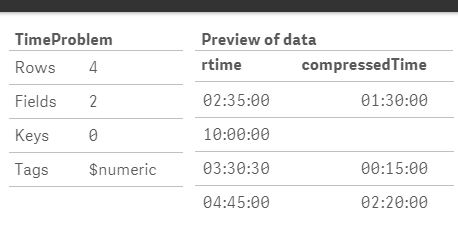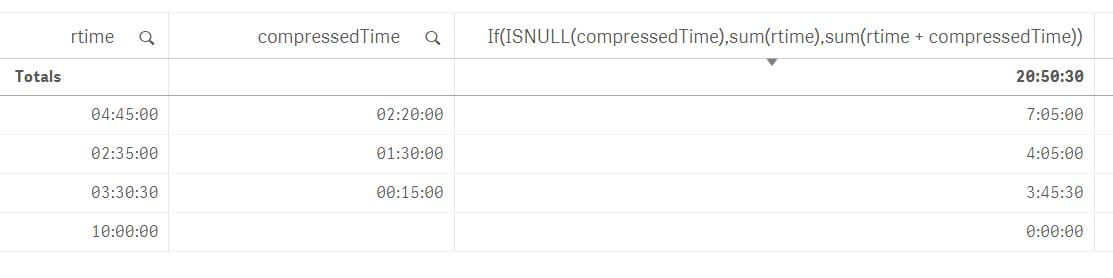# Qlik Sense App Development

Discussion board where members can learn more about Qlik Sense App Development and Usage.

Announcements
Qlik® Product Spotlight: Discover what’s possible. Get more from our products.
See for yourself. Register today.
Not applicable

## Calculate sum if field exists

I need to update my load script to perform the following.  I have two fields one which is always assigned to a record (Time) and a second field (compressedTime) which could exist on the record.

1)  Check if a field exists (compressedTime) on the record and calculate the sum.

2)  If the field DOES NOT exist on the record then calculate the sum of another field (Time).

3)  Sum of the two fields based on the above criteria.

1 Solution

Accepted Solutions
Not applicable

## Re: Calculate sum if field exists

Try:

If(ISNULL(compressedTime), Time, compressedTime) AS CurrectTime

AND then you can sum the calculated field (CurrectTime).

7 Replies
Not applicable

## Re: Calculate sum if field exists

In both fields - is the non exists values defined as NULL?

Not applicable

## Re: Calculate sum if field exists

Unfortunately no - we use a non-relational DB, so the field either exists on the record or not.  We don't insert this field on every record and then assign a NULL value if empty.

Not applicable

## Re: Calculate sum if field exists

I can't understand your data structure, are the fields in different tables?

Can you please share your original table so I can help more?

Not applicable

## Re: Calculate sum if field exists

All the fields are in the same table.

Not applicable

## Re: Calculate sum if field exists

Try:

If(ISNULL(compressedTime), Time, compressedTime) AS CurrectTime

AND then you can sum the calculated field (CurrectTime).

Not applicable

## Re: Calculate sum if field exists

I think this is what you want.  If the compressed time is there you want to sum record time and compressed time otherwise just sum the record time.

TimeProblem:

load * Inline [

rtime, compressedTime

02:35:00, 01:30:00

10:00:00,

03:30:30, 00:15:00

04:45:00, 02:20:00

];Not applicable

## Re: Calculate sum if field exists

Thanks for the feedback Mary!

Community Browser